# The Only Guide You Need: ISERROR Excel

Jul 14, 2023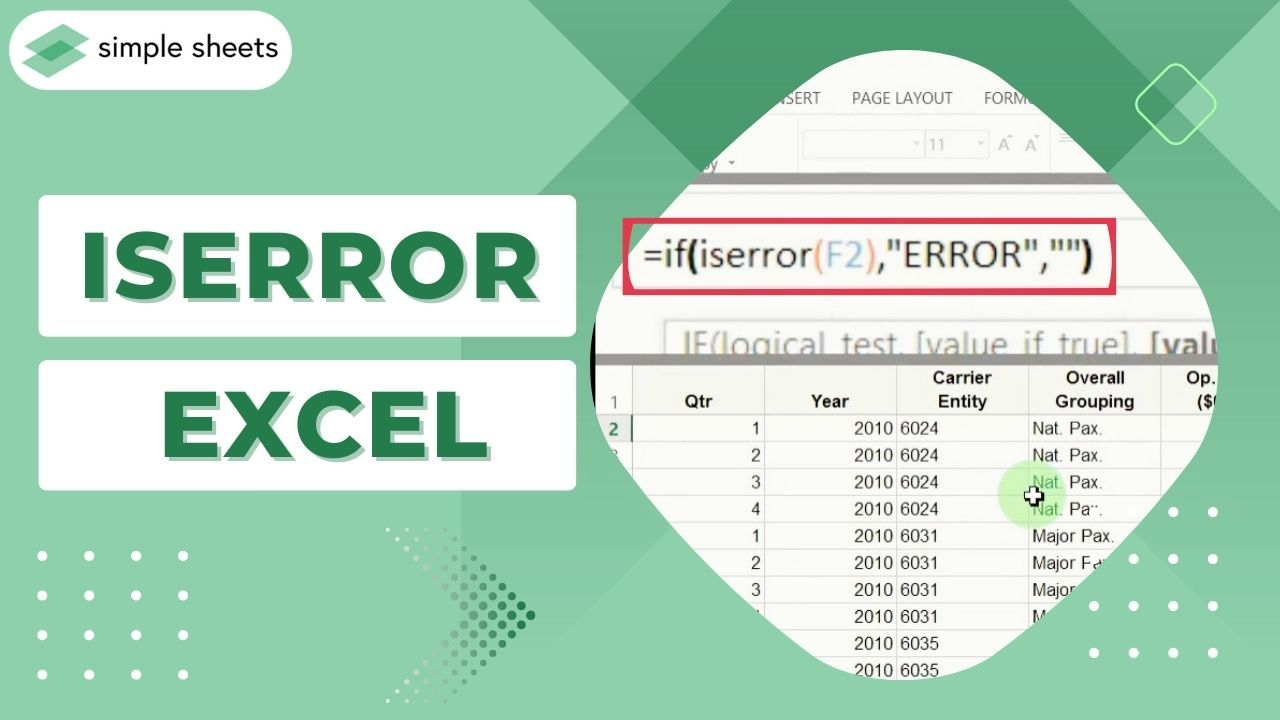Do you ever feel like Excel is out to get you?

Anyone who has spent time debugging spreadsheet formulas can relate to this problem. The dreaded Excel errors can be frustrating and lead to incorrect results if not fixed in time.

Thankfully, an Excel function called ISERROR can help take the guesswork out of debugging formula issues.

In this blog post, we'll discuss the ISERROR function and how it can effectively troubleshoot errors in your spreadsheets.

Read on as we cover the following:

• What Is the ISERROR Function in Excel?

• IF and ISERROR Functions in Excel

• VLOOKUP and IF ISERROR Functions in Excel

• Final Thoughts on the ISERROR Excel Function

• Frequently Asked Questions on the ISERROR Excel Function## What Is the ISERROR Function in Excel?

The Excel ISERROR function can identify all kinds of errors, including #CALC!, #DIV/0!, #N/A, #NAME?, #NUM!, #NULL!, #REF!, #VALUE!, and #SPILL!.

Additionally, this feature returns  If an error occurs, you'll get a "TRUE" value. Otherwise, the function will give a "FALSE" value.

### ISERROR function syntax in Excel

The syntax for the ISERROR function is straightforward, as illustrated in the image below.

• Value - the cell reference.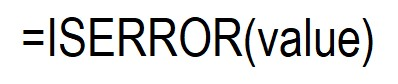### Learn to use the ISERROR function.

To use the ISERROR function in Excel, follow the steps below: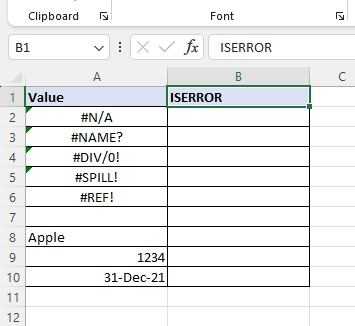1. Type the ISERROR formula with its lookup value in a blank cell.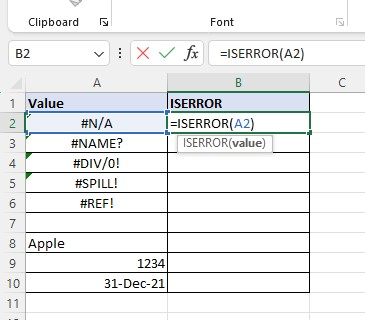1. Press the Enter key.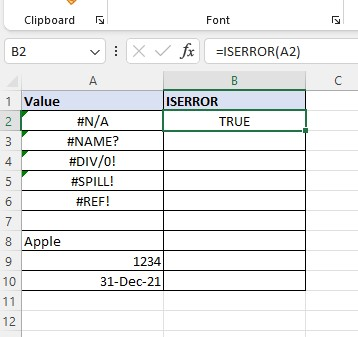1. Use the fill handle to fill the other rows.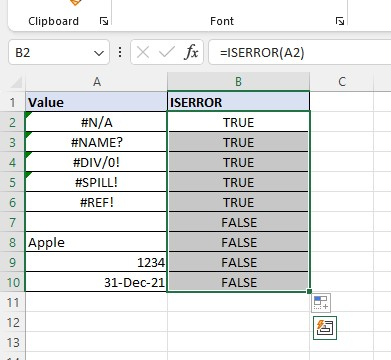In the example above, ISERROR returns a TRUE value if it detects an error message. Meanwhile, the ISSEROR function returns a FALSE value if it doesn't detect an error.

## IF and ISERROR Functions in Excel

If you want to retrieve a custom message when an error occurs in your spreadsheet, use the ISERROR function with the IF function.

To use the IF and ISERROR functions, follow the formula below: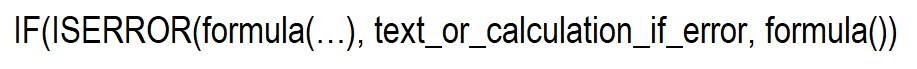### Learn to use the IF with ISERROR functions.

1. Prepare your data with errors.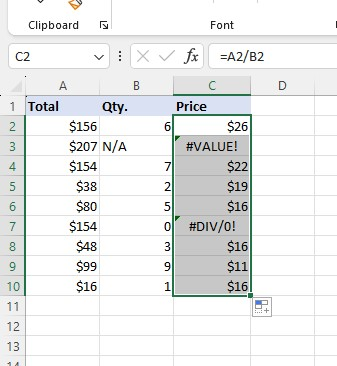1. To replace error messages with custom messages, use the IF and ISERROR functions.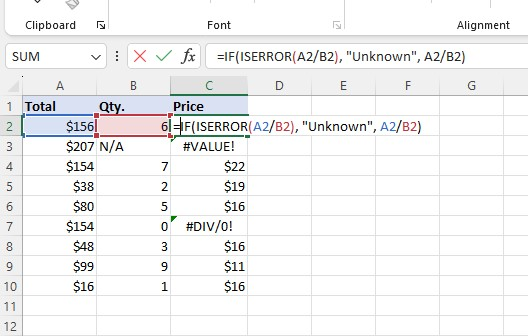1. Press the Enter key. As you will notice, it returns the same value but has a different formula.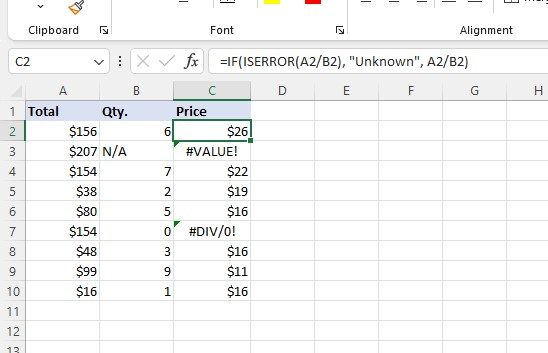1. Use the auto-fill feature to apply the formula for the remaining rows.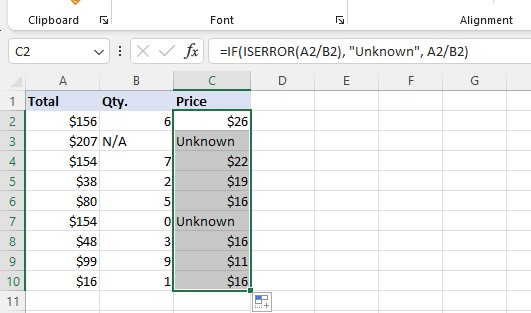You will notice in the last step that the error messages turn into a custom message "Uknown" in Column C.

## VLOOKUP and IF ISERROR Functions in Excel

If your VLOOKUP formula can't find the lookup value, use the IF ISERROR functions with the VLOOKUP function.

VLOOKUP formula With IF ISERROR functions syntax: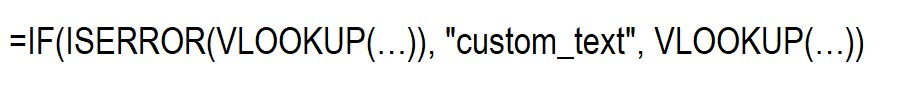### Learn to use the VLOOKUP with IF ISERROR functions.

1. Prepare your data with a lookup table.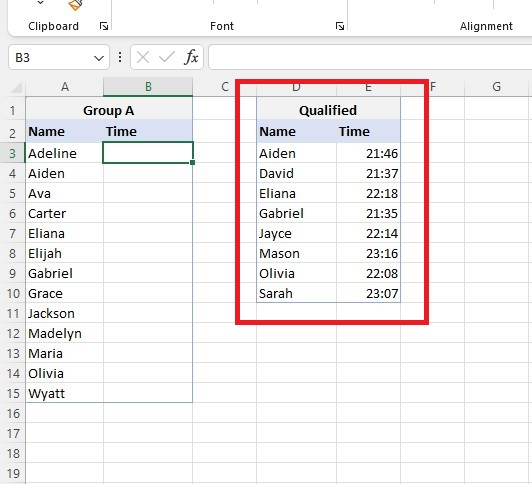1. Type the VLOOKUP with the IF ISERROR formula.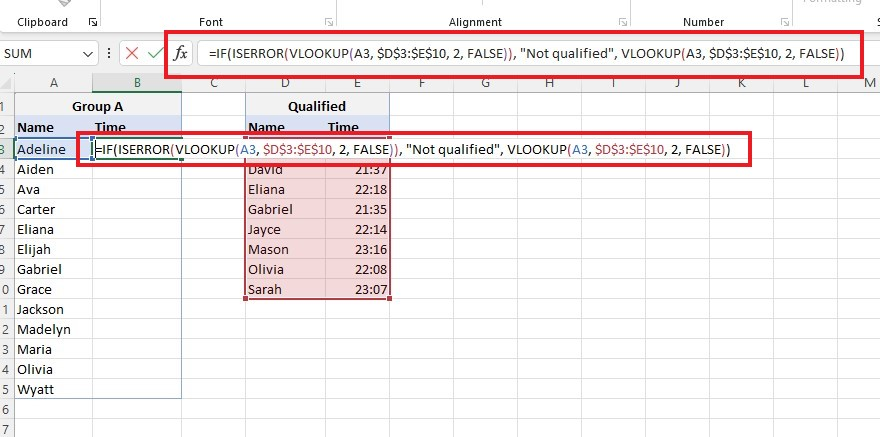1. Press Enter to get the result of the formula.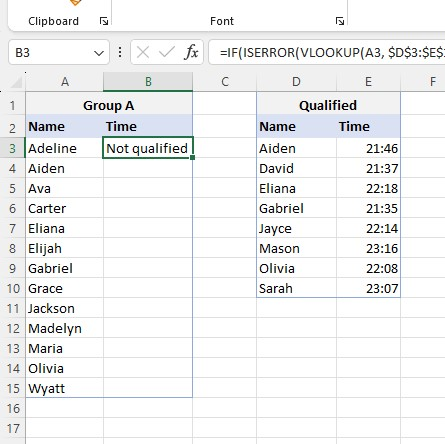1. Fill the other rows using h the auto-fill feature.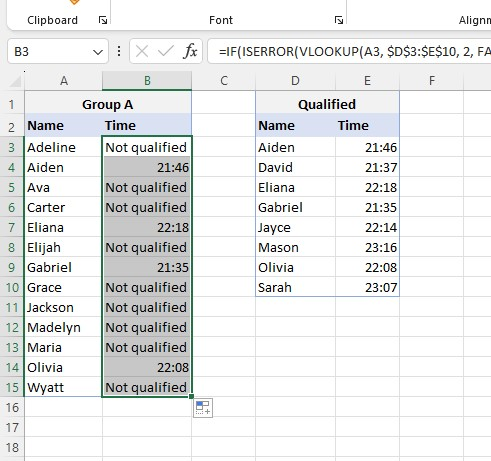If the data you seek is not in the table, the output will be "Not qualified."

## Final Thoughts on the ISERROR Excel Function

ISERROR can be an incredibly powerful and useful function for overcoming various data problems in Excel.

Learning how this tool works may take practice, but your effort will eventually pay off.

Visit Simple Sheets for more easy-to-follow Excel guides, and remember to visit the related articles section of this blog post.

For the most straightforward Excel video tutorials, subscribe to Simple Sheets on YouTube!## Frequently Asked Questions on ISERROR Excel

### How are the IFERROR and ISERROR functions different?

The IFERROR function allows providing a substitute value or action when encountering errors. Meanwhile, you can use the ISERROR function to verify if a value or formula is incorrect.

### How can I count the errors from the ISERROR function in a column?

You use the SUMPRODUCT or SUM function to count the number of errors.

Additionally, press the CTRL+SHIFT+ENTER keys to create an array formula for the SUM and ISERROR functions to work.

### What would happen if I only used the VLOOKUP function instead of the IF ISERROR formula?

The VLOOKUP function returns an error message instead of a custom message if it doesn't find a value in the lookup table.## Related Articles

The Excel IFERROR Function: What is it and When to Use it

Excel Array Formulas: What Is It, And How to Use It

#### Want to Make Excel Work for You? Try out 5 Amazing Excel Templates & 5 Unique Lessons

We hate SPAM. We will never sell your information, for any reason.